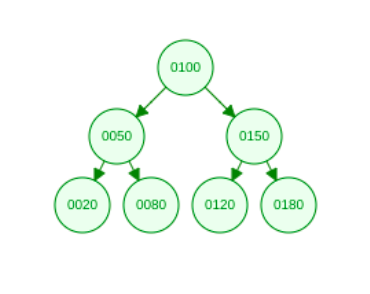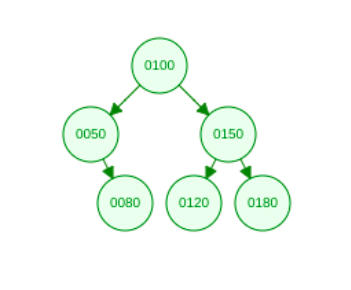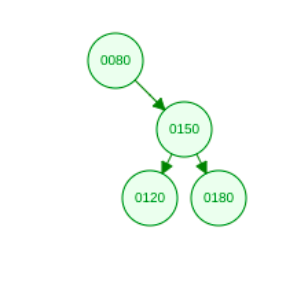# Binary Search Trees (BST) - III

##### Deleting a node from a given BSTBatoi Research Group Jun 27, 2021

In this article, we will discuss deleting a node from a given binary search tree. Before we delete a node, we have to know what kind of node is it. A BST can have three types of nodes:1. Node with no child, i.e., leaf node. We simply remove this kind of node from the tree—for example, 20.1. Node with one child: We replace this node with its child node (left or right). For example, 501. Node with two children: We replace its contents with a minimum value in its right subtree or maximum value in its left subtree for this kind of node. And delete that minVal/maxVal node.

This can also be rephrased as replacing the contents of the node to be deleted with the inorder successor (node that comes just after the node to be deleted in the inorder traversal), which will be the minimum value in the right subtree or replacing contents of the node to be deleted with the inorder predecessor (node that comes just before the node to be deleted in the inorder traversal) which will be the maximum value in the left subtree — and deleting the inorder successor/predecessor node after; for example, 100.CODE:


#include
using namespace std;

class TreeNode{
public:
int val;
TreeNode *left, *right;
TreeNode(int data){
val = data;
left = right = NULL;
}
};

void inorder(TreeNode* root){
if(!root) return;
inorder(root->left);
coutleft = insert(root->left, val);
else
root->right = insert(root->right, val);
return root;
}

TreeNode* minValueNode(TreeNode* root){
TreeNode* temp = root;
while(temp->left)
temp = temp->left;
return temp;
}
TreeNode* deleteTreeNode(TreeNode* root, int val){
if (root == NULL)
return root;

if (val < root->val)
root->left = deleteTreeNode(root->left, val);

else if (val > root->val)
root->right = deleteTreeNode(root->right, val);

else {
if (root->left == NULL && root->right == NULL)
return NULL;

else if (root->left == NULL) {
TreeNode* temp = root->right;
free(root);
return temp;
}
else if (root->right == NULL) {
TreeNode* temp = root->left;
free(root);
return temp;
}

TreeNode* temp = minValueNode(root->right);
root->val = temp->val;
root->right = deleteTreeNode(root->right, temp->val);
}
return root;

}
int main()
{
/* Let us create the following BST
100
/     \
50      150
/  \    /  \
20   80  120   180 */
TreeNode* root = NULL;
root = insert(root, 100);
root = insert(root, 50);
root = insert(root, 150);
root = insert(root, 20);
root = insert(root, 80);
root = insert(root, 120);
root = insert(root, 180);

cout

### Need our assistance? We are available with you.